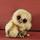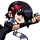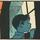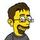## General Question# Did I find the diagonal of a rectangle correctly?

Asked by chelle21689 (7883) November 27th, 2010

The diagonal of a rectangle is 25 cm.
a. Find the dimensions of the rectangle if it is 5 cm longer than it is wide.
w=width
w+(w+5)^2=25^2

w=15

w+5=20

Question b. What are the dimensions if the rectangle is a square?

s=length of each side
s^2+s^2=25^2
s^2=625/2

That’s how far I got and I’m confused what the answer might be. I don’t know how to get to that final step. Please help.

Observing members: 0Composing members: 0Take the square root of both sides.

Ivan (13479)“Great Answer” (2) Flag as…I did do that which leaves me with s= sq rt 625/2

I don’t know the answer…it doesn’t ask to round or anything so I’m not sure. Square root of 625/2….am I supposed to reduce or something? Someone told me it was s= 25 square root 2

chelle21689 (7883)“Great Answer” (0) Flag as…It’s s = 25/(sqrt2).

The diagonal and two of the sides form a 45–45-90 triangle. In any such triangle, the diagonal is always equal to the side length times sqrt2. So if the diagonal is 25, then the side lengths are 25/sqrt2.

Your method works just as well. When you take the square root of 625/2, you take the square root of the numerator and denominator separately. The square root of 625 is 25, so the square root of 625/2 is 25/sqrt2, just like we found using the other method.

Ivan (13479)“Great Answer” (1) Flag as…@Ivan it.s not a square- the length is 5cm longer than the width Pythagoras says a^2+b^2=c^2 or
25^2 = w^2 + (w+5)^2

you can figure it out from there.

zenvelo (37454)“Great Answer” (0) Flag as…@zenvelo

I’m discussing part b.

Ivan (13479)“Great Answer” (1) Flag as…the basic length of the hypotenuse of a square relating to the sides is a multiple of square root of 2. Think of a 1×1 square.so you’re on the right track with 2s^2=625.

zenvelo (37454)“Great Answer” (0) Flag as…but then when you have 2^s2=625
you get s^2=625/2

then square root both sides
then you get

S= square root 625/2

and then I’m stuck. Do I just hit square root 625/2 on my calc and approximate the answer?

do I try to simplify??

chelle21689 (7883)“Great Answer” (0) Flag as…It looks to me like something isn’t quite right there…

w+(w+5)^2=25^2

Correct me if I am wrong, but it seems to me that that first one should read:

(w^2) + ((w+5)^2) = 25^2

Was that a typo?

As for the second one, I would simplify it first;

s^2 = 625/2—> s^2 = 312.5

jerv (31061)“Great Answer” (1) Flag as…yeah typo lol

chelle21689 (7883)“Great Answer” (0) Flag as…Who cares what format you have the answer in. The point is that you know how to solve it, and you have the correct answer. I would leave it as simply 25/sqrt2, but that’s just me.

Ivan (13479)“Great Answer” (0) Flag as…The exact form of the answer depends on what form your teacher expects. Usually, 25/sqrt2 should be simplified. It is generally preferred not to have a square root in the denominator. To take care of that, multiply both the numerator and denominator by sqrt2 to get 25sqrt2/2.

LostInParadise (29657)“Great Answer” (0) Flag as…Most of my math and science teachers wanted the simplest answer possible, so the form does matter quite a bit. Hell, there have been many times where I got the correct answer and got only 1 point on a 5 point question since the method matters more than the final answer!

That is why I went with sqrt(312.5), though most teachers I’ve had would want that evaluated/calculated to an actual number. In this case, I think rounding to 17.7 would be acceptable as it’s close to the correct answer (17.67766952966…) and, more importantly, it shows that you have the math skills to actually do it right.

jerv (31061)“Great Answer” (0) Flag as…thank you every one

chelle21689 (7883)“Great Answer” (0) Flag as…or